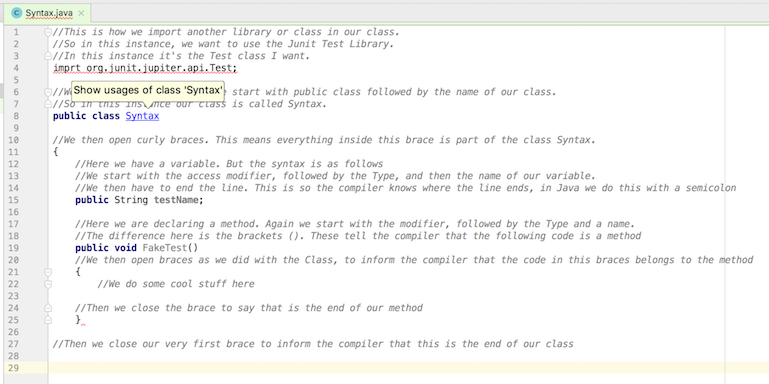# Java Syntax

As mentioned in the programming basics course, each programming language has a specific syntax. A specific way to write code so that the compiler can compile it.

Here are some code and comments to explain the basic Syntax of Java.

``````//This is a code comment

//This is how we import another library or class in our class.
//So in this instance, we want to use the JUnit Test Library.
//In this instance, it's the Test class I want.
import org.junit.Test;

//We need to open our class. We start with public class followed by the name of our class.
//So in this instance, our class is called Syntax.
public class Syntax

//We then open curly braces. This means everything inside this brace is part of the class Syntax.
{
//Here we have a variable. But the syntax is as follows
//We start with the access modifier, followed by the Type, and then the name of our variable.
//We then have to end the line. This is so the compiler knows where the line ends, in Java we do this with a semicolon
public String testName;

//Here we are declaring a method. Again we start with the modifier, followed by the Type and a name.
//The difference here is the brackets (). These tell the compiler that the following code is a method
public void FakeTest()
//We then open braces as we did with the Class, to inform the compiler that the code in this braces belongs to the method
{
//We do some cool stuff here

//Then we close the brace to say that is the end of our method
}

//Then we close our very first brace to inform the compiler that this is the end of our class
}

``````

The nice thing about using an IDE is that if you get this syntax wrong it will usually tell you. So in IntelliJ, it will make the class red and put a squiggly red line under what it believes to be the cause.In this example, I’ve added a typo in ‘import’ at the top, and I’ve removed the curly brace that closes the class. There is a lot more syntax in Java, but this should be enough to get you going.

### Next Lesson ➔Author